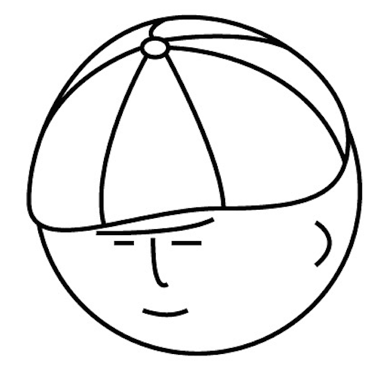# マツシタのお勉強メモ

#### マツシタのお勉強

##### welcome to my engineering blog
###### Hacker Rank# Find the heavy bottle

Problem You have 20 bottles of pills. 19 bottles have 1.0 gram pills, but one has pills, but one has pills of weight 1.1 grams. Given a scale that provides an exact measurement, how would you find the heavy bottle? You can only use the sca…

# Create a Linked List of all the nodes at each depth in Binary Tree

Problem Given a binary tree, design a algorithm which creates a linked list of all the nodes at each depth (e.g, if you have a tree with depth D, you'll have D linked lists) How to Solve The important point of the this problem How we shoul…

# Make a Binary Search Tree from sorted array

Problem Given a sorted (increasing order) array with unique integer elements, write an algorithm to create a binary search tree with minimal height. How to Solve This problem asks me to create a BST (Binary Search Tree) from array. What is…

# Find out whether there is a routes between two nodes

Problem Given a directed graph, design an algorithm to find out whether there is a route between two nodes. How to Solve This problem can be solved by depth first search or breadth first search. While traversing the graph, we have to contr…

# Check if a binary tree is balanced

Problem Implement a function to check if a binary tree is balanced. For the purposes of this question. balanced tree is defined to be a tree such that the heights of the two subtrees of any node never differ by more than one. How to Solve …